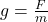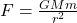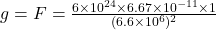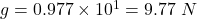## how to find a planet’s gravitational field strength using its radius? solve: the radius of earth is 6.4 x 10^6 m and its mass is

Question

how to find a planet’s gravitational field strength using its radius?
solve:
the radius of earth is 6.4 x 10^6 m and its mass is 6.0 x 10^24. calculate earth’s gravitational field strength.

in progress 0
3 months 2021-08-22T11:37:30+00:00 1 Answers 1 views 0

1. The gravitational field strength is approximately equal to 10 N.

Explanation:

Gravitational field strength is the measure of gravitational force acting on any object placed on the surface of the planet. Generally, the mass of the object is considered as 1 kg.

So the gravitational field strength will be equal to the gravitational force acting on the object.

The formula for gravitational field strength isHere g is the gravitational field strength, m is the mass of the object placed on the surface and F is the gravitational force acting on the object.

Since, the mass of any object placed on the surface of earth will be negligible compared to the mass of Earth, so the mass of the object is considered as 1 kg.

Then the g = F

AndHere G is the gravitational constant, M is the mass of Earth and m is the mass of the object placed on the surface, while r is the radius of the Earth.So, the gravitational field strength is approximately equal to 10 N.